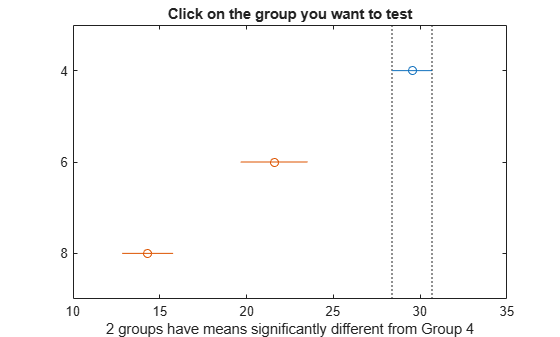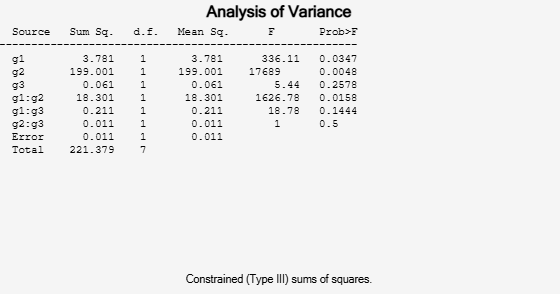## Multiple Comparisons

### Introduction

Analysis of variance (ANOVA) techniques test whether a set of group means (treatment effects) are equal or not. Rejection of the null hypothesis leads to the conclusion that not all group means are the same. This result, however, does not provide further information on which group means are different.

Performing a series of t-tests to determine which pairs of means are significantly different is not recommended. When you perform multiple t-tests, the probability that the means appear significant, and significant difference results might be due to large number of tests. These t-tests use the data from the same sample, hence they are not independent. This fact makes it more difficult to quantify the level of significance for multiple tests.

Suppose that in a single t-test, the probability that the null hypothesis (H0) is rejected when it is actually true is a small value, say 0.05. Suppose also that you conduct six independent t-tests. If the significance level for each test is 0.05, then the probability that the tests correctly fail to reject H0, when H0 is true for each case, is (0.95)6 = 0.735. And the probability that one of the tests incorrectly rejects the null hypothesis is 1 – 0.735 = 0.265, which is much higher than 0.05.

To compensate for multiple tests, you can use multiple comparison procedures. The Statistics and Machine Learning Toolbox™ function `multcompare` performs multiple pairwise comparison of the group means, or treatment effects. The options are Tukey’s honestly significant difference criterion (default option), the Bonferroni method, Scheffe’s procedure, Fisher’s least significant differences (lsd) method, and Dunn & Sidák’s approach to t-test.

To perform multiple comparisons of group means, provide the structure `stats` as an input for `multcompare`. You can obtain `stats` from one of the following functions :

For multiple comparison procedure options for repeated measures, see `multcompare` (`RepeatedMeasuresModel`).

### Multiple Comparisons Using One-Way ANOVA

`load carsmall`

`MPG` represents the miles per gallon for each car, and `Cylinders` represents the number of cylinders in each car, either 4, 6, or 8 cylinders.

Test if the mean miles per gallon (mpg) is different across cars that have different numbers of cylinders. Also compute the statistics needed for multiple comparison tests.

```[p,~,stats] = anova1(MPG,Cylinders,'off'); p```
```p = 4.4902e-24 ```

The small p-value of about 0 is a strong indication that mean miles per gallon is significantly different across cars with different numbers of cylinders.

Perform a multiple comparison test, using the Bonferroni method, to determine which numbers of cylinders make a difference in the performance of the cars.

`[results,means] = multcompare(stats,'CType','bonferroni')````results = 3×6 1.0000 2.0000 4.8605 7.9418 11.0230 0.0000 1.0000 3.0000 12.6127 15.2337 17.8548 0.0000 2.0000 3.0000 3.8940 7.2919 10.6899 0.0000 ```
```means = 3×2 29.5300 0.6363 21.5882 1.0913 14.2963 0.8660 ```

In the `results` matrix, 1, 2, and 3 correspond to cars with 4, 6, and 8 cylinders, respectively. The first two columns show which groups are compared. For example, the first row compares the cars with 4 and 6 cylinders. The fourth column shows the mean mpg difference for the compared groups. The third and fifth columns show the lower and upper limits for a 95% confidence interval for the difference in the group means. The last column shows the p-values for the tests. All p-values are zero, which indicates that the mean mpg for all groups differ across all groups.

In the figure the blue bar represents the group of cars with 4 cylinders. The red bars represent the other groups. None of the red comparison intervals for the mean mpg of cars overlap, which means that the mean mpg is significantly different for cars having 4, 6, or 8 cylinders.

The first column of the `means` matrix has the mean mpg estimates for each group of cars. The second column contains the standard errors of the estimates.

### Multiple Comparisons for Three-Way ANOVA

```y = [52.7 57.5 45.9 44.5 53.0 57.0 45.9 44.0]'; g1 = [1 2 1 2 1 2 1 2]; g2 = {'hi';'hi';'lo';'lo';'hi';'hi';'lo';'lo'}; g3 = {'may';'may';'may';'may';'june';'june';'june';'june'};```

`y` is the response vector and `g1`, `g2`, and `g3` are the grouping variables (factors). Each factor has two levels, and every observation in `y` is identified by a combination of factor levels. For example, observation `y(1)` is associated with level 1 of factor `g1`, level `'hi'` of factor `g2`, and level `'may'` of factor `g3`. Similarly, observation `y(6)` is associated with level 2 of factor `g1`, level `'hi'` of factor `g2`, and level `'june'` of factor `g3`.

Test if the response is the same for all factor levels. Also compute the statistics required for multiple comparison tests.

```[~,~,stats] = anovan(y,{g1 g2 g3},'model','interaction',... 'varnames',{'g1','g2','g3'});```The p-value of 0.2578 indicates that the mean responses for levels `'may'` and `'june'` of factor `g3` are not significantly different. The p-value of 0.0347 indicates that the mean responses for levels `1` and `2` of factor `g1` are significantly different. Similarly, the p-value of 0.0048 indicates that the mean responses for levels `'hi'` and `'lo'` of factor `g2` are significantly different.

Perform multiple comparison tests to find out which groups of the factors `g1` and `g2` are significantly different.

`results = multcompare(stats,'Dimension',[1 2])````results = 6×6 1.0000 2.0000 -6.8604 -4.4000 -1.9396 0.0272 1.0000 3.0000 4.4896 6.9500 9.4104 0.0170 1.0000 4.0000 6.1396 8.6000 11.0604 0.0136 2.0000 3.0000 8.8896 11.3500 13.8104 0.0101 2.0000 4.0000 10.5396 13.0000 15.4604 0.0087 3.0000 4.0000 -0.8104 1.6500 4.1104 0.0737 ```

`multcompare` compares the combinations of groups (levels) of the two grouping variables, `g1` and `g2`. In the `results` matrix, the number 1 corresponds to the combination of level `1` of `g1` and level `hi` of `g2`, the number 2 corresponds to the combination of level `2` of `g1` and level `hi` of `g2`. Similarly, the number 3 corresponds to the combination of level `1` of `g1` and level `lo` of `g2`, and the number 4 corresponds to the combination of level `2` of `g1` and level `lo` of `g2`. The last column of the matrix contains the p-values.

For example, the first row of the matrix shows that the combination of level `1` of `g1` and level `hi` of `g2` has the same mean response values as the combination of level `2` of `g1` and level `hi` of `g2`. The p-value corresponding to this test is 0.0280, which indicates that the mean responses are significantly different. You can also see this result in the figure. The blue bar shows the comparison interval for the mean response for the combination of level `1` of `g1` and level `hi` of `g2`. The red bars are the comparison intervals for the mean response for other group combinations. None of the red bars overlap with the blue bar, which means the mean response for the combination of level `1` of `g1` and level `hi` of `g2` is significantly different from the mean response for other group combinations.

You can test the other groups by clicking on the corresponding comparison interval for the group. The bar you click on turns to blue. The bars for the groups that are significantly different are red. The bars for the groups that are not significantly different are gray. For example, if you click on the comparison interval for the combination of level `1` of `g1` and level `lo` of `g2`, the comparison interval for the combination of level `2` of `g1` and level `lo` of `g2` overlaps, and is therefore gray. Conversely, the other comparison intervals are red, indicating significant difference.

### Multiple Comparison Procedures

To specify the multiple comparison procedure you want `multcompare` to conduct use the `'CType'` name-value pair argument. `multcompare` provides the following procedures:

#### Tukey’s Honestly Significant Difference Procedure

You can specify Tukey’s honestly significant difference procedure using the `'CType','Tukey-Kramer'` or `'CType','hsd'` name-value pair argument. The test is based on studentized range distribution. Reject H0:αi = αj if

`$|t|=\frac{|{\overline{y}}_{i}-{\overline{y}}_{j}|}{\sqrt{MSE\left(\frac{1}{{n}_{i}}+\frac{1}{{n}_{j}}\right)}}>\frac{1}{\sqrt{2}}{q}_{\alpha ,k,N-k,}$`

where ${q}_{\alpha ,k,N-k}$ is the upper 100*(1 – α)th percentile of the studentized range distribution with parameter k and Nk degrees of freedom. k is the number of groups (treatments or marginal means) and N is the total number of observations.

Tukey’s honestly significant difference procedure is optimal for balanced one-way ANOVA and similar procedures with equal sample sizes. It has been proven to be conservative for one-way ANOVA with different sample sizes. According to the unproven Tukey-Kramer conjecture, it is also accurate for problems where the quantities being compared are correlated, as in analysis of covariance with unbalanced covariate values.

#### Bonferroni Method

You can specify the Bonferroni method using the `'CType','bonferroni'` name-value pair. This method uses critical values from Student’s t-distribution after an adjustment to compensate for multiple comparisons. The test rejects H0:αi = αj at the $\alpha /2\left(\begin{array}{l}k\\ 2\end{array}\right)$ significance level, where k is the number of groups if

`$|t|=\frac{|{\overline{y}}_{i}-{\overline{y}}_{j}|}{\sqrt{MSE\left(\frac{1}{{n}_{i}}+\frac{1}{{n}_{j}}\right)}}>{t}_{\alpha }{2\left(\begin{array}{c}k\\ 2\end{array}\right)},N-k,}$`

where N is the total number of observations and k is the number of groups (marginal means). This procedure is conservative, but usually less so than the Scheffé procedure.

#### Dunn & Sidák’s Approach

You can specify Dunn & Sidák’s approach using the `'CType','dunn-sidak'` name-value pair argument. It uses critical values from the t-distribution, after an adjustment for multiple comparisons that was proposed by Dunn and proved accurate by Sidák. This test rejects H0:αi = αj if

`$|t|=\frac{|{\overline{y}}_{i}-{\overline{y}}_{j}|}{\sqrt{MSE\left(\frac{1}{{n}_{i}}+\frac{1}{{n}_{j}}\right)}}>{t}_{1-\eta /2,v,}$`

where

`$\eta =1-{\left(1-\alpha \right)}^{{}^{1}{\left(\begin{array}{l}k\\ 2\end{array}\right)}}}$`

and k is the number of groups. This procedure is similar to, but less conservative than, the Bonferroni procedure.

#### Least Significant Difference

You can specify the least significance difference procedure using the `'CType','lsd'` name-value pair argument. This test uses the test statistic

`$t=\frac{{\overline{y}}_{i}-{\overline{y}}_{j}}{\sqrt{MSE\left(\frac{1}{{n}_{i}}+\frac{1}{{n}_{j}}\right)}}.$`

It rejects H0:αi = αj if

`$|{\overline{y}}_{i}-{\overline{y}}_{j}|>\underset{LSD}{\underbrace{{t}_{\alpha }{2},N-k}\sqrt{MSE\left(\frac{1}{{n}_{i}}+\frac{1}{{n}_{j}}\right)}}}.$`

Fisher suggests a protection against multiple comparisons by performing LSD only when the null hypothesis H0: α1 = α2 = ... = αk is rejected by ANOVA F-test. Even in this case, LSD might not reject any of the individual hypotheses. It is also possible that ANOVA does not reject H0, even when there are differences between some group means. This behavior occurs because the equality of the remaining group means can cause the F-test statistic to be nonsignificant. Without any condition, LSD does not provide any protection against the multiple comparison problem.

#### Scheffe’s Procedure

You can specify Scheffe’s procedure using the `'CType','scheffe'` name-value pair argument. The critical values are derived from the F distribution. The test rejects H0:αi = αj if

`$\frac{|{\overline{y}}_{i}-{\overline{y}}_{j}|}{\sqrt{MSE\left(\frac{1}{{n}_{i}}+\frac{1}{{n}_{j}}\right)}}>\sqrt{\left(k-1\right){F}_{k-1,N-k,\alpha }}$`

This procedure provides a simultaneous confidence level for comparisons of all linear combinations of the means. It is conservative for comparisons of simple differences of pairs.

 Milliken G. A. and D. E. Johnson. Analysis of Messy Data. Volume I: Designed Experiments. Boca Raton, FL: Chapman & Hall/CRC Press, 1992.

 Neter J., M. H. Kutner, C. J. Nachtsheim, W. Wasserman. 4th ed. Applied Linear Statistical Models.Irwin Press, 1996.

 Hochberg, Y., and A. C. Tamhane. Multiple Comparison Procedures. Hoboken, NJ: John Wiley & Sons, 1987.